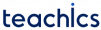In this tutorial, you will learn the breadth first search (BFS) algorithm for traversing a graph data structure with examples.

Depth-first search or depth-first traversal is a recursive algorithm used to visit all the vertices in a graph.

In the BFS algorithm, the traversal starts from a node and then carries onto its adjacent nodes. It traverses all the sibling nodes within a level and then moves to the next level. The search continues until all the vertices are visited.

BFS implementation puts each vertex in the graph into the following lists:

• Visited.
• Not Visited (Queue).

This marking is used to avoid cycles while traversing. The BFS algorithm performs the following operations:

1. Put the starting node to the back of the Queue.
2. Repeat Steps 3 and 4 until Queue is empty.
3. Remove the front node N of Queue and add it to the Visited List.
4. Add all unvisited neighbours of N to the rear of the Queue.

#### Depth First Search Algorithm Example

Consider the following graph with 5 vertices.

Start from vertex A, put A on the visited list and add all its adjacent vertices to the queue.

Now visit the element at the FRONT of the queue (B). Go to unvisited adjacent vertex (C) of B.

Visit D which is at the front of the queue.

Now only E remains in the queue and all other adjacent nodes are already visited.

#### Applications of Breadth First Search Algorithm

• Finding all connected components in a graph G.
• Finding the shortest path between two unweighted network nodes, u and v.
• Finding the shortest path between two weighted network nodes, u and v
Subscribe
Notify of